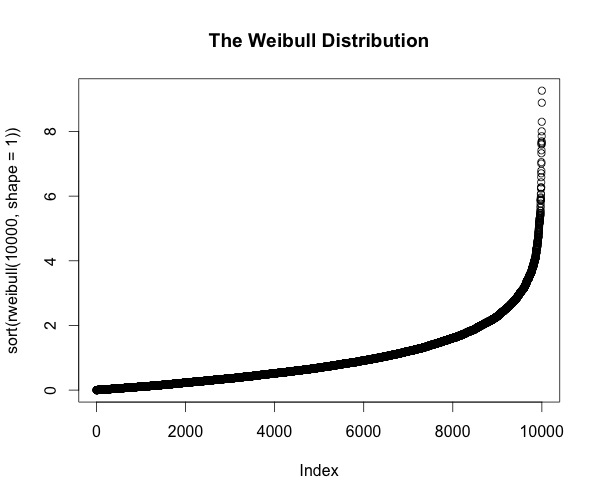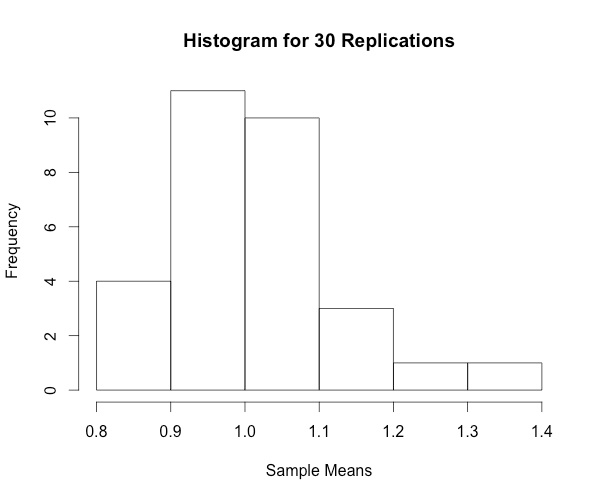# A Brief Exercise Illustrating the Central Limit Theorem

Posted by Mischa Fisher in Econometrics
Thu 26 November 2015

Succinctly, the Central Limit Theorem can be expressed as:

In probability theory, the central limit theorem (CLT) states that, given certain conditions, the arithmetic mean of a sufficiently large number of iterates of independent random variables, each with a well-defined expected value and well-defined variance, will be approximately normally distributed, regardless of the underlying distribution.

If you're an econometrics student, it's likely the first time you're exposed to the CLT is when discussing the OLS estimator in the context of testing hypothesis about the true parameters in order to form confidence intervals: when relying on asymptotics, the normality of the error distributions is not required because as long as the normal 5 Gauss-Markov assumptions are satisfied, the distribution of the OLS estimator will converge to a normal distribution as n goes to infinity.

The CLT is pretty neat and is given short shrift in the context of econometrics, so, here's a brief experiment one can perform in R to illustrate what happens as the theorem comes into effect.

Starting with the Weibull distribution:

plot(sort(rweibull(10000, shape=1)), main="The Weibull Distribution")We then sample the means of the distribution in increasing replications, then draw histograms from the distributions.

hist(colMeans(replicate(30,rweibull(100,shape=1))),breaks="Scott", xlab="Sample Means", main="Histogram for 30 Replications")
hist(colMeans(replicate(300,rweibull(100,shape=1))),breaks="Scott", xlab="Sample Means", main="Histogram for 300 Replications")
hist(colMeans(replicate(3000,rweibull(100,shape=1))),breaks="Scott", xlab="Sample Means", main="Histogram for 3,000 Replications")
hist(colMeans(replicate(30000,rweibull(100,shape=1))),breaks="Scott", xlab="Sample Means", main="Histogram for 30,000 Replications")
hist(colMeans(replicate(300000,rweibull(100,shape=1))),breaks="Scott", xlab="Sample Means", main="Histogram for 300,000 Replications")
hist(colMeans(replicate(3000000,rweibull(100,shape=1))),breaks="Scott", xlab="Sample Means", main="Histogram for 3,000,000 Replications")


Revealing, a very wonderful .gif :As the replication size increases, the histogram begins to resemble a normal distribution. Neat!

### UPDATE:

A friend writes:

You should emphasize more the process that you are taking the MEAN of sub samples of your 'population' which is Weibull distributed. Then, by creating a vector of these means, one is able to show that these "means" converge to a normal distribution as N approaches infinity. As it is, to the 'less' experienced reader perhaps will fail to realize that you take the means of sub samples of that pop'n, and then it is the means which become normally distributed.

Good point; thanks Keith!